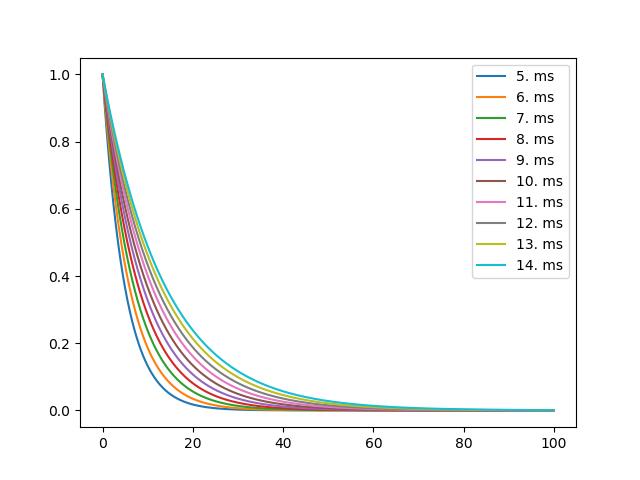Example: 02_using_standalone¶

Note

You can launch an interactive, editable version of this example without installing any local files using the Binder service (although note that at some times this may be slow or fail to open):Parallel processes using standalone mode

This example use multiprocessing to run several simulations in parallel. The code is using the C++ standalone mode to compile and execute the code.

The generated code is stored in a standalone{pid} directory, with pid being the id of each process.

Note that the set_device() call should be in the run_sim function.

By moving the set_device() line into the parallelised function, it creates one C++ standalone device per process. The device.reinit() needs to be called` if you are running multiple simulations per process (there are 10 tau values and num_proc = 4).

Each simulation uses it’s own code folder to generate the code for the simulation, controlled by the directory keyword to the set_device call. By setting directory=None, a temporary folder with random name is created. This way, each simulation uses a different folder for code generation and there is nothing shared between the parallel processes.

If you don’t set the directory argument, it defaults to directory="output". In that case each process would use the same files to try to generate and compile your simulation, which would lead to compile/execution errors.

Setting directory=f"standalone{pid}" is even better than using directory=None in this case. That is, giving each parallel process it’s own directory to work on. This way you avoid the problem of multiple processes working on the same code directories. But you also don’t need to recompile the entire project at each simulation. What happens is that in the generated code in two consecutive simulations in a single process will only differ slightly (in this case only the tau parameter). The compiler will therefore only recompile the file that has changed and not the entire project.

The numb_proc sets the number of processes. run_sim is just a toy example that creates a single neuron and connects a StateMonitor to record the voltage.

For more details see the discussion in the Brian forum.

import os
import multiprocessing
from time import time as wall_time
from os import system
from brian2 import *

def run_sim(tau):
pid = os.getpid()
directory = f"standalone{pid}"
set_device('cpp_standalone', directory=directory)
print(f'RUNNING {pid}')

G = NeuronGroup(1, 'dv/dt = -v/tau : 1', method='euler')
G.v = 1

mon = StateMonitor(G, 'v', record=0)
net = Network()
net.run(100 * ms)
res = (mon.t/ms, mon.v)

device.reinit()

print(f'FINISHED {pid}')
return res

if __name__ == "__main__":
start_time = wall_time()

num_proc = 4
tau_values = np.arange(10)*ms + 5*ms
with multiprocessing.Pool(num_proc) as p:
results = p.map(run_sim, tau_values)

print(f"Done in {wall_time() - start_time:10.3f}")

for tau_value, (t, v) in zip(tau_values, results):
plt.plot(t, v, label=str(tau_value))
plt.legend()
plt.show()